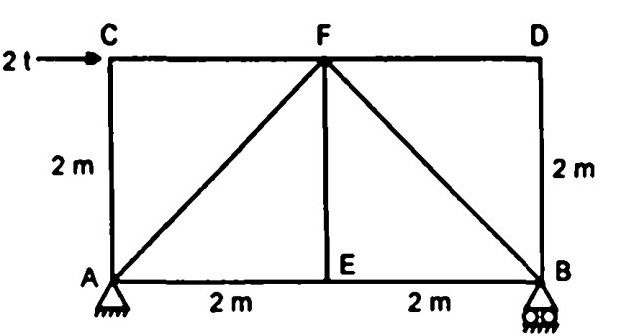top of pageSearch

# Strength of Materials(301-320)

Updated: Apr 29, 2020

301.A straight wire 15 m long is subjected to a tensile stress of 2000 kgf/cm2. Elastic modulus is 1.5 x 106 kgf/cm². Coefficient of linear expansion for the material is 16.66 x 10⁻⁶/°F. The temperature change in °F) to produce the same elongation as due to the 2000 kgf/cm² tensile stress in the material is 80

302. System A is a simply supported beam with a load P at mid span. System B is the same beam but the load is replaced by a udl of intensity P/L wherein L is the span. The mid span deflection of system B will

be less than that of system A at mid span

303. Given that

PE = the cripping load given by Euler,

Pc = the load at failure due to direct compression

PR = the load in accordance with the

Rankine's criterion of failure,

PR is given by304. A simply supported truss shown in Fig. carries load as shown. The force in

member BE is1 t (tensile)

305. The modular ratio of the materials used in the flitched beam is 10 and the ratio of the allowable stresses is also 10. Four different sections of the beam are shown in Fig. The material shown hatched has larger modulus of elasticity and allowable stress than the rest.Section II would support the greatest load

306. A column ABCD of rectangular section is subjected to an eccentric load at Z as shown in Fig.Under the compressive load, the direct stress is 400 t/m² and bending stresses are

σₓₓ = ± 1200 t/m² and σᵧᵧ = ± 800 t/m² The stress at the corner A will be

800 t/m² (compressive)

307. The plane of maximum shear stress has normal stress that is none

308. In the analysis of a closely coiled helical spring under axial load, which of the

following is /are negligible ?

1. Bending

2. Axial force in the wire

309. A thick cylinder is subjected to external pressure. The magnitude of hoop stress at internal radius will be greater than the magnitude of hoop stress at external radius

310. A composite section made of two materials has moduli of elasticity in the

ratio 1: 2 and lengths in the ratio 2:1. The ratio of corresponding stresses under a

311.The slope at the support A of the overhanging beam shown in Fig. is

Wl²/24EI

312. The material of a rubber balloon has Poisson's ratio of 0.5. If uniform pressure

is applied to blow the balloon, the volumetric strain of the material will be zero

313. Sets of principal stresses acting at any point in a stressed body are given below: The correct sequence of the ascending order of intensity of the maximum shear stress induced by the above sets will be314.Consider the following statements regarding a simply supported beam subjected to a uniformly distributed load over the entire span:

1. The bending moment is maximum at the central position.

2. The shear force is zero at the central position

315316.317. A simply supported beam of uniform cross section is subjected to a maximum

bending moment of 2.25 t.m. If it has rectangular cross section with width 15

cm and depth 30 cm, then the maximum bending stress induced in the beam will be 100 kg/cm²

318. A beam has a solid circular cross section having diameter d. If a section of the

beam is subjected to a shear force, F, the maximum shear stress in the cross section is given by319.A cantilever beam 'A' with rectangular cross section is subjected to a

concentrated load at its free end. If width and depth of another cantilever beam 'B' are twice those of beam A, then the deflection at free end of the beam 'B' as

compared to that of 'A' will be 6.25%

320.Consider a close coiled helical spring of radius R, subjected to a load. P. The

spring consists of n turns of wire with wire radius r. The stiffness of the spring

is